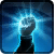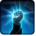CZ-Dark Control FlareConditions

Related effects, buffs and debuffs

Please click on an effect below to view its details.

• [does not expire]CZ-Dark Control Flare

 Slot: Buff Duration: passive Tick rate: does not tick Initial stacks: 1 Max stacks: 100 # occurrences: 1 Tags: 6851971988964066167, 16620541020863806165
• When effect is applied

Perform the following actions:

• Play appearance epp . operation . dxun . boss . holding_pens . player . grant_flare . buff, dependent on calling effect
• Unknown (167)
- Lock Out Player Abilities = (bool) false
- Temporary Package Spec = (int) 16141159339814348152
• Custom
- Param 2 = (string) Creating new Flare Charge owner
- Param 3 = (string)
- Param 4 = (string)
- Param 5 = (string)
- Param 6 = (string)
- Param 7 = (string)
- Param 8 = (string)
- Param 9 = (string)
- Param 1 = (int) 9499481598613081908
• Just before NPC with this effect dies
• [target override]

Perform the following actions:

• Unknown (164)
- Ability Spec = (int) 16141118710142653929
- From Actor = (int) 2
- To Actor = (int) 2
• Custom
- Param 2 = (string) Flare Charge holder has died, Execute Drop Unlit Flares ability
- Param 3 = (string)
- Param 4 = (string)
- Param 5 = (string)
- Param 6 = (string)
- Param 7 = (string)
- Param 8 = (string)
- Param 9 = (string)
- Param 1 = (int) 9499481598613081908
• Just before this effect is removed

Perform the following actions:

• Custom
- Param 2 = (string) Flare Charge parent effect removed, cleaning up all associated children
- Param 3 = (string)
- Param 4 = (string)
- Param 5 = (string)
- Param 6 = (string)
- Param 7 = (string)
- Param 8 = (string)
- Param 9 = (string)
- Param 1 = (int) 9499481598613081908
• Dispel 99 effects
• When TARGET gets revived

Perform the following actions:

• Dispel 99 effects
• Custom
- Param 2 = (string) We've been revived! Terminate flare granting AOE!
- Param 3 = (string)
- Param 4 = (string)
- Param 5 = (string)
- Param 6 = (string)
- Param 7 = (string)
- Param 8 = (string)
- Param 9 = (string)
- Param 1 = (int) 9499481598613081908
• When NPC with this effect receives an effect with tags 4890358484277421296
• [target override]

Only when the following conditions are met:

• <
• If TARGET is not alive
AND
• If TARGET has an effect with the tag 6851971988964066167
>

Perform the following actions:

• Custom
- Param 2 = (string) Flare charge request acknowledged, processing Grant Flare to Trigger Caster
- Param 3 = (string)
- Param 4 = (string)
- Param 5 = (string)
- Param 6 = (string)
- Param 7 = (string)
- Param 8 = (string)
- Param 9 = (string)
- Param 1 = (int) 9499481598613081908
• Modify Charges
- Ability Spec = (int) 0
- Amount Max = (float) -1
- Amount Min = (float) -1
- Amount Percent = (float) 0
• Add effect #2 to TARGET from PRIMARY TARGET
• When stacks of this effect decrease

Only when the following conditions are met:

• If stacks of this effect = 0

Perform the following actions:

• Custom
- Param 2 = (string) Flare charge count as 0, requesting removal
- Param 3 = (string)
- Param 4 = (string)
- Param 5 = (string)
- Param 6 = (string)
- Param 7 = (string)
- Param 8 = (string)
- Param 9 = (string)
- Param 1 = (int) 9499481598613081908
• Remove this effect
• [0s] [not displayed]Effect #2

 Slot: Buff Duration: 0s Tick rate: does not tick # occurrences: 0 Tags: 16620541020863806165 Conditions: Can only by called by other effects
• On Apply

Only when the following conditions are met:

• If PRIMARY TARGET does not have any effect with the tag 16620541020863806165

Perform the following actions:

• Unknown (164)
- Ability Spec = (int) 16141175915292831245
- From Actor = (int) 3
- To Actor = (int) 3
• Custom
- Param 2 = (string) Flare request acknowledged, Executing Grant Ability
- Param 3 = (string)
- Param 4 = (string)
- Param 5 = (string)
- Param 6 = (string)
- Param 7 = (string)
- Param 8 = (string)
- Param 9 = (string)
- Param 1 = (int) 9499481598613081908
• On Apply

Only when the following conditions are met:

• If PRIMARY TARGET has an effect with the tag 16620541020863806165

Perform the following actions:

• Modify Charges
- Ability Spec = (int) 0
- Amount Max = (float) 1
- Amount Min = (float) 1
- Amount Percent = (float) 0
• Custom
- Param 2 = (string) Flare request acknowledged, incrementing Flare Charge by 1
- Param 3 = (string)
- Param 4 = (string)
- Param 5 = (string)
- Param 6 = (string)
- Param 7 = (string)
- Param 8 = (string)
- Param 9 = (string)
- Param 1 = (int) 9499481598613081908